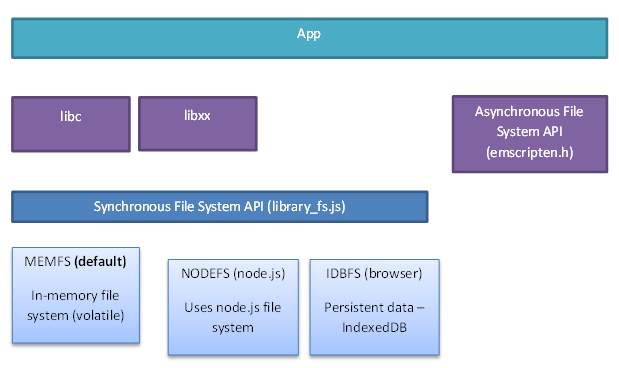# Emscripten的文件系统

## Overview## 使用示例

### C程序

```#include <stdio.h>

void append_line(char *filename, char *line) {
FILE *fp = fopen(filename, "a");
fprintf(fp, "%s\n", line);
fclose(fp);
}

int main() {
return 0;
}
```

### 编译

```emcc fs.c \
-o fs.js \
-s EXPORTED_RUNTIME_METHODS="[cwrap, FS]" \
-s EXPORTED_FUNCTIONS="[_append_line]"
```

```<body>
<script src="fs.js"></script>
<script>
const appendLine = Module.cwrap('append_line', null, ['string', 'string'])
</script>
</body>
```

### 使用

```> Module.FS.readFile('/tmp/test.txt', { encoding: 'utf8' })
"line1\n"
```

## Emscripten文件系统的实现

### WebAssembly调用JavaScript代码

```(import "env" "__sys_open" (func \$env.__sys_open (type \$t1)))
(import "wasi_snapshot_preview1" "fd_close" (func \$wasi_snapshot_preview1.fd_close (type \$t0)))
(import "env" "__sys_fcntl64" (func \$env.__sys_fcntl64 (type \$t1)))
(import "env" "__sys_ioctl" (func \$env.__sys_ioctl (type \$t1)))
(import "wasi_snapshot_preview1" "fd_write" (func \$wasi_snapshot_preview1.fd_write (type \$t9)))
(import "env" "__sys_mkdir" (func \$env.__sys_mkdir (type \$t2)))
(import "env" "emscripten_resize_heap" (func \$env.emscripten_resize_heap (type \$t0)))
(import "env" "emscripten_memcpy_big" (func \$env.emscripten_memcpy_big (type \$t1)))
(import "env" "setTempRet0" (func \$env.setTempRet0 (type \$t4)))
(import "wasi_snapshot_preview1" "fd_seek" (func \$wasi_snapshot_preview1.fd_seek (type \$t7)))
...
```

```return fetch(wasmBinaryFile, { credentials: 'same-origin' }).then(function (response) {
var result = WebAssembly.instantiateStreaming(response, info);
...
})
```

```var asmLibraryArg = {
"__sys_fcntl64": ___sys_fcntl64,
"__sys_ioctl": ___sys_ioctl,
"__sys_open": ___sys_open,
"emscripten_memcpy_big": _emscripten_memcpy_big,
"emscripten_resize_heap": _emscripten_resize_heap,
"fd_close": _fd_close,
"fd_seek": _fd_seek,
"fd_write": _fd_write,
"setTempRet0": _setTempRet0
};

var info = {
'env': asmLibraryArg,
'wasi_snapshot_preview1': asmLibraryArg,
};
```

### JavaScript实现

Emscripten虚拟文件系统的实现位于源码的 `src` 目录下，主体是 `library_fs.js`，各个具体实现分别位于 `library_memfs.js`, `library_idbfs.js` 等。

```readdir: function(path) {
var lookup = FS.lookupPath(path, { follow: true });
var node = lookup.node;
throw new FS.ErrnoError({{{ cDefine('ENOTDIR') }}});
}
}
```

```FSNode {
id         // 一个自增的数字
parent     // 指向节点的父节点（root节点的父节点是自己）
mode       // 节点的类型及读写模式
name       // 文件名或目录名
contents   // 文件内容，如果节点是目录则是目录下文件名到子节点的map
node_ops   // 节点操作
}
```

```readdir: function(node) {
var entries = ['.', '..'];
for (var key in node.contents) {
if (!node.contents.hasOwnProperty(key)) {
continue;
}
entries.push(key);
}
return entries;
}
```

```readdir: function(node) {
var path = NODEFS.realPath(node);
try {